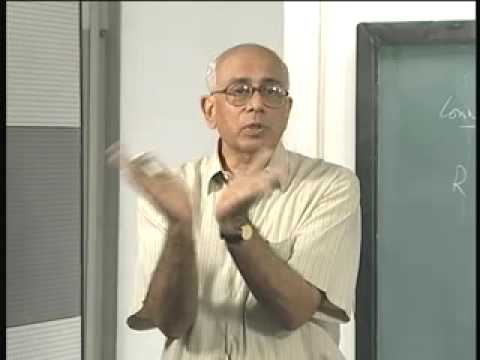NPTEL Courses

# Quantum Physics

The course covers topics in quantum physics: Heisenberg's uncertainty principle, linear vector spaces, linear operations, Hamiltonian of the system, Schrodinger equation, Hermite polynomials, probability density, eigenvalues and eigenstates of the Hamiltonian, spin, simple harmonic oscillators, properties of spinning particles, orbital angular momentum, Pauli matrices, the energy of the vacuum, and perturbation theory. (from nptel.ac.in )

Instructor

Physics
2009

#### Lecture 24 - Equivalence Class